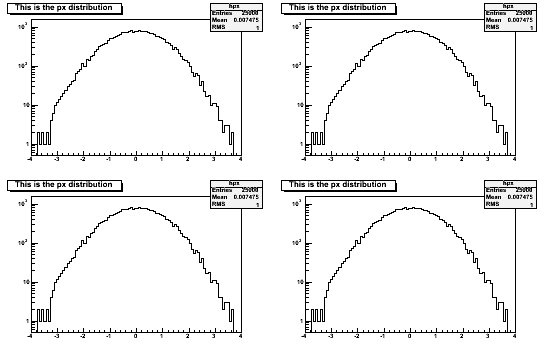# Setlogy

Hi! I’d like to know how I can use SetLogy when I have a loop as in the followiong example:

TCanvas *c1 = new TCanvas(“c1”,“canvas”,800,600);

``````c1->Divide(2,2);

for (int m=0;m<4;m++){
c1->cd(m+1);
}

c1->Print(title,"gif");
c1->Update();
c1->Clear();
``````

It seems that gPad doesn’t work

``````{
TCanvas *c1 = new TCanvas("c1","canvas",800,600);
c1->Divide(2,2);
for (int i = 1; i<=4 ; i++) {
c1->cd(i);
hpx->Draw();```for (int m=0;m<4;m++){ TVirtualPad *pad = c1->cd(m+1); pad->SetLogy(); hCha_Rate_PAD_CM_Ijk_Sect7[l][m]->Draw(); } ```